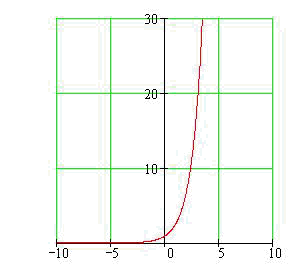Calculus on Demand at Dartmouth College Lecture 16 | Index | Lecture 18 Lecture 17Resources

Math 3 Course Syllabus
Practice Exams

Contents

In this lecture we discuss modeling with differential equations, the unifying concept of lectures 17–20. We also take a first look at a class of differential equations called "separable."

Quick Question

What function Q satisfies the differential equation dQ/dt = et?Outline

Outlines for Modeling with Differential Equations

Textbook

Modeling with Differential Equations; Separable Differential Equations: First Look